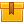# Introduction to Algebra (A4306)

## Prerequisites

This lesson is designed to build on the skills presented in the fifth lesson of this program Positive and Negative Numbers, Powers, and Roots.

## Description

This lesson introduces algebra by presenting typical symbols that are used inmathematical expressions. In this lesson, participants learn how to write and solve equations.

## Objectives

Define the term variable. Substitute symbols for words in mathematical expressions. Combine like terms in a mathematical expression. Use unit analysis to estimate answers to mathematical equations. State the rules for adding and subtracting like and unlike terms. State the rules for multiplying and dividing like and unlike terms. Define the term equation. Given word problem, write the corresponding equation. Recognize common equations (formulas). Use common equations to solve problems. Estimate the answers to problems involving variables. Manipulate equations to solve for an unknown variable. Identify the proper order of operations. Use positive and negative signs in equations. Use powers and roots in equations; solve equations. Identify key words in the problem. Solve a multiple-equation problem.

## ContentThis lesson introduces algebra by presenting typical symbols that are
used in mathematical expressions. In this lesson, participants learn how
to write and solve equations.

•Introduction to Algebra

0
0 Reviews